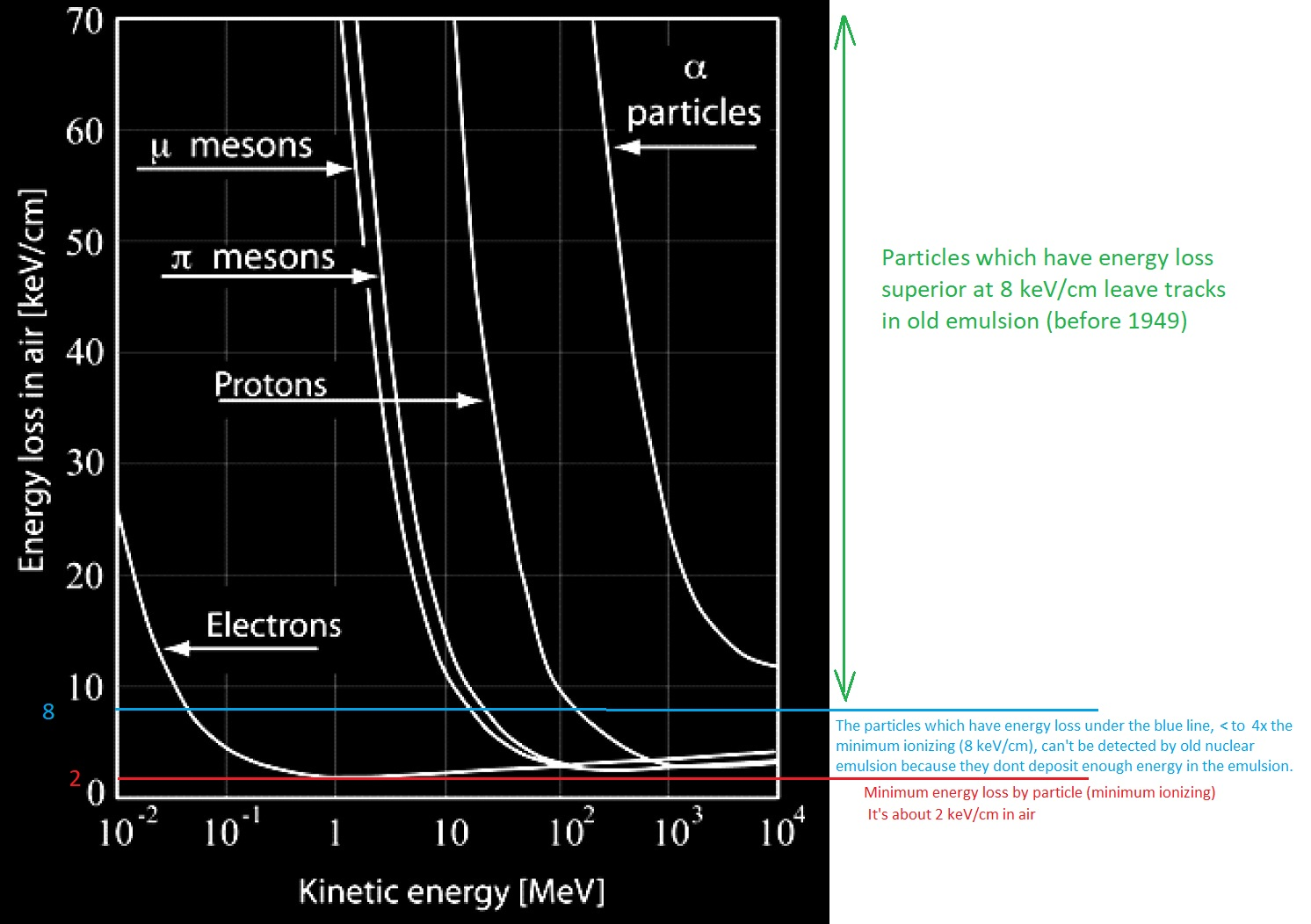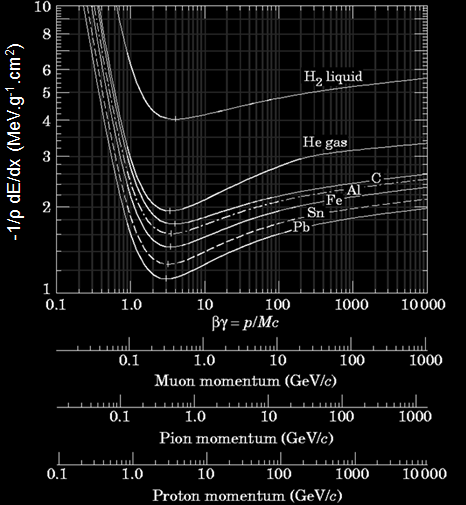## short explanationNote : the minimum ionizing value is the least for electrons. So it require very sensitive photographic emulsion capable to record the lowest deposit of energy (keV/cm).

When a particle travel in matter, it loose a mean energy in keV/cm. The curve show the variation of energy loss with the kinetic energy of particle. As the particle slow down, it loose a huge amount of energy in matter.  But if ths particle have a lot of energy (travelling near the speed of light), it loose few energy per cm.

For example, an electron of 10 keV loose about 25 keV/cm in air, it’s speed is about 60.000 km/s.

At a certain point of the curve, the energy loss is minimal. For a electron, this occurs at 1 MeV, when it’s speed is about 282.000 km/s. At this point it loose only 2 keV/cm in air. Then the energy loss increase slightly with the increasing speed of electron.

For heavier and singly charged particle, the minimum ionisation is roughly between 10 to 100 MeV.

Under 10 MeV, theses particles loose a hugh amount of energy per cm, it’s exponential.

The problem with the emulsion before 1949 is that they are sensitive only to particles about 4x time the minimum ionisation (so in air, that’s about 4×2 = 8 keV/cm). So fast particles for example a proton of 500 MeV, don’t leave track in the emulsion. So there is a threshold of detection for non minimum ionizing sensitive emulsion and they can’t be use for the study of interactions of fast particles (specially cosmic rays which have great kinetic energy).

The curve above is specifically for air. For other material, we have to consider this curve :This curve is the average energy loss (ΔE) for a particle of charge e (proton, electron, muon…but not alpha as e=2). It’s interesting to note that the energy loss is roughly equal to 2 MeV.cm2/g for solid or gaseous material. Density of he gas is 10 x time less than air (air is 0.00123 g/cm3).

To obtain the linear energy loss (MeV/cm) in a medium of density ρ,  just multiply ΔE by ρ.

For example in air we will choose the He gas curve. The minimum ionization is 2 MeV.cm²/g. So the linear energy loss in air is 2 MeV.cm2/g x 0.00123 g/cm3 = 0,00246 MeV/cm => 2,4 keV/cm. Previous curve in top of the page gave 2 keV/cm.

Emulsion of silver halide have a density about 4 g/cm3 . So the minimum average energy loss is 1.5 MeV.cm2/g (we take the middle between Al and Fe curve which have a density about 2.7 and 7.8 g/cm3. So at minimum ionisation, the specific ionisation of a particle of charge e in photographic emulsion is 1,5 x 4 = 6 MeV/cm or 600 keV/mm. In the literature of 1952, it’s 700 keV/mm. The number of grains in the track of a particle of charge e at minimum ionisation in a typical well developed emulsion is ~270 per mm.